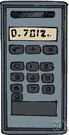# calculator

(redirected from Calculater)
Also found in: Thesaurus, Encyclopedia.
Related to Calculater: Scientific calculator

## cal·cu·la·tor

(kăl′kyə-lā′tər)
n.
1. One that calculates, as:
a. An electronic or mechanical device for the performance of mathematical computations.
b. A person who operates such a machine or otherwise makes calculations.
2. A set of mathematical tables used to aid in calculating.

## calculator

(ˈkælkjʊˌleɪtə)
n
1. (Mathematics) a device for performing mathematical calculations, esp an electronic device that can be held in the hand
2. a person or thing that calculates
3. (Mathematics) a set of tables used as an aid to calculations

## cal•cu•la•tor

(ˈkæl kyəˌleɪ tər)

n.
1. a small, hand-operated electronic or mechanical device that performs calculations.
2. a set of tables that facilitate calculation.
3. a person who calculates.
[1375–1425]
ThesaurusAntonymsRelated WordsSynonymsLegend:
 Noun 1calculator - an expert at calculation (or at operating calculating machines)expert - a person with special knowledge or ability who performs skillfullyadder - a person who adds numbersnumber cruncher - someone able to perform complex and lengthy calculationsactuary, statistician - someone versed in the collection and interpretation of numerical data (especially someone who uses statistics to calculate insurance premiums)subtracter - a person who subtracts numbers 2calculator - a small machine that is used for mathematical calculationscalculating machineabacus - a calculator that performs arithmetic functions by manually sliding counters on rods or in groovesadder - a machine that adds numbersadding machine, totaliser, totalizer - a calculator that performs simple arithmetic functionstabulator, counter - a calculator that keeps a record of the number of times something happenshand calculator, pocket calculator - a calculator small enough to hold in the hand or carry in a pocketmachine - any mechanical or electrical device that transmits or modifies energy to perform or assist in the performance of human tasksNapier's bones, Napier's rods - a set of graduated rods formerly used to do multiplication and division by a method invented by John Napierquipu - calculator consisting of a cord with attached cords; used by ancient Peruvians for calculating and keeping recordssubtracter - a machine that subtracts numbers
Translations
آلَةٌ حَاسِبَةحاسِب، حاسِبَه، عَدّاد
kalkulačkapočítačka
kalkulator
számológépzsebszámológép
reiknivél, tölva

계산기
calculator
kalkulačka
kalkulator
računalo
miniräknareräknarekalkylator
เครื่องคิดเลข
hesap makinesihesap makinası
máy tính

## calculator

[ˈkælkjʊleɪtəʳ] N (= machine) →

## calculator

[ˈkælkjʊleɪtər] n
(also pocket calculator) →
(on computer)
(= formula) →

## calculator

n
(= machine)Rechner m
(= person)Kalkulator(in) m(f), → Rechnungsbeamte(r) m/-beamtin f
(= table of figures)Rechentabelle f

## calculator

[ˈkælkjʊˌleɪtəʳ] n

## calculate

(ˈkӕlkjuleit) verb
to count or estimate, using numbers. Calculate the number of days in a century.